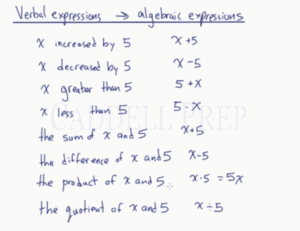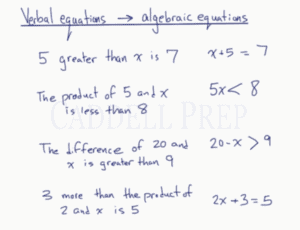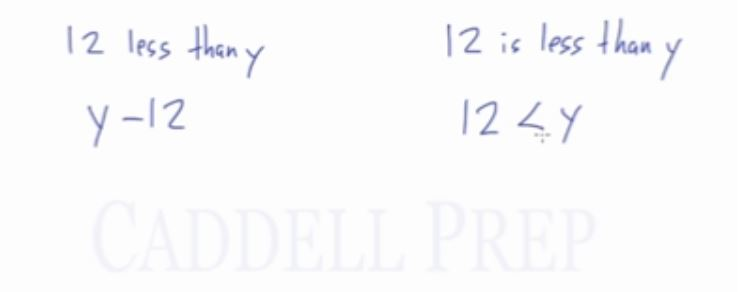In this video, we are going to learn how to turn verbal expressions and equations into algebraic expressions and equations. When turning expressions into mathematical expressions, we use keywords to determine the operation that should be used. Specific keywords or phrases in the verbal expression allows us to also determine the order of numbers and variables, “greater than”, “less than”, and “difference of”. After you finish this lesson, view all of our Pre-Algebra and Algebra 1 lessons and practice problems.

## Verbal Expressions to Algebraic Expressions

x increased by 5$\rightarrow x+5$
x decreased by 5$\rightarrow x-5$
x less than 5$\rightarrow 5-x$### Verbal Equations to Algebraic Equations## Examples of Verbal To Algebraic Expressions & Equations

### Example 1

The product of$10$ and$b$ decreased by$2$

The product of$10$ and$b$ would be$10 \times b$ which we know is$10x$.

Decreased means we subtract.

Therefore, the algebraic expression is,$10b-2$

### Example 2

The quotient of$28$ and$p$ is less than$10$

Since quotient is the answer to division, it would be$28 \div p$

The less than sign is$\textless$

Therefore,$28 \div p \textless 10$

## Video-Lesson Transcript

Let’s go over changing verbal expression into algebraic expressions.

For example:$x$ increased by$5$ this will be$x + 5$.$x$ decreased by$5$ since it’s a decrease, we are taking$5$ away from$x$. So this becomes$x - 5$.$x$ greater than$5$, here we have$x$ more than$5$. We have$5$ and we’re increasing it by$x$. Here we have$5 + x$.$x$ less than$5$. So think about this, if we have$2$ less than$5$. We have$5$ and we’re taking$2$ away from it. So it’s$5 - x$.

The sum of$x$ and$5$ is just$x + 5$.

The difference of$x$ and$5$, difference is the answer to subtraction so we have is$x - 5$.

Then the product of$x$ and$5$ would be$x \times5$ which we know is$5x$.

The order isn’t important in multiplication and addition.

The quotient of$x$ and$5$, since quotient is the answer to division our answer would be$x \div5$.

You should get familiar with these different expressions. Some may memorize these. But it’s nice to look at these expressions and make sense of it.

Now, let’s look at changing verbal equations to algebraic equations.

Verbal equations are expressions that have equal signs.

So, we’re going to do this in equality. Like equal to, less than, greater than, less than or equal to, or greater than or equal to.

Let’s have some examples.$5$ greater than$x$ is$7$.

This becomes$x + 5 = 7$.

The product of$5$ and$x$ is less than$8$.$5x \textless 8$

The difference of$20$ and$x$ is greater than$9$.$20 -x \textgreater 9$$3$ more than the product of$2$ and$x$ is$5$.$2x + 3 = 5$

Let me point out the difference between these two:$12$ less than$y$
and$12$ is less than$y$$12$ less than$y$ is$y - 12$

While$12$ is less than$y$ is$12 \textless y$.

“Is” in the sentence is an equality sign. That’s why we come up with the less than sign.